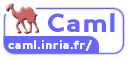# OCaml

Implementation of programming language OCaml

The official OCaml implementation.OCaml Inria logo

## Examples:

### Hello, World!:

Example for versions OCaml 3.11

`print_endline` is a built-in function defined with the following type:

``````string -> unit = <func>
``````

This means that it takes 1 string as a parameter, and returns the unit type, `()`.

``````let () = print_endline "Hello World";;
``````

### Factorial:

Example for versions OCaml 3.11

This example uses an auxiliary function `fact`, so that tail recursion is possible.

``````let rec fact n accum =
if n <= 1 then
accum
else
fact (n-1) (accum*n);;

let factorial n =
fact n 1;;

let () =
for n = 0 to 16 do
Printf.printf "%d! = %d\n" n (factorial n)
done;
``````

### Factorial:

Example for versions OCaml 3.11

This example shows the naive way to implement the factorial function. However, it is not tail recursive, since the recursive function call is not the only statement on the line.

``````let rec factorial n =
if n <= 1 then
1
else
factorial (n-1) * n;;

let () =
for n = 0 to 16 do
Printf.printf "%d! = %d\n" n (factorial n)
done;
``````

### Fibonacci numbers:

Example for versions OCaml 3.11

This example uses straightforward recursive solution. `Printf.printf` does formatted output.

``````let rec fibonacci n =
if n < 3 then
1
else
fibonacci (n-1) + fibonacci (n-2)

let () =
for n = 1 to 16 do
Printf.printf "%d, " (fibonacci n)
done;
print_endline "..."
``````

Example for versions OCaml 3.11

``````let square x = x *. x;;

let delta  a b c = ( square b-. (4. *. a *. c ));;

let   solve a b c   =
if a=0.
then Printf.printf "Not a quadratic equation\n"
else
if   delta a b c  >= 0.
then
let   x1=( -.b +. sqrt(delta a b c )) /. (2. *. a)
and x2=  (-.b -.  sqrt(delta a b c ))  /. (2. *. a)
in
Printf.printf "x1 =%.5f x2=%.5f \n"  x1  x2
else
let   x= (-.b   /. (2. *. a))
and i=     sqrt(4. *. a *. c -. square b)  /. (2. *. a)
in Printf.printf "x+ =%.5f+i%.5f  x-=%.5f-i%.5f \n"  x  i  x i
;;

let () =
solve (float_of_string Sys.argv.(1))( float_of_string Sys.argv.(2) )(float_of_string Sys.argv.(3))
``````